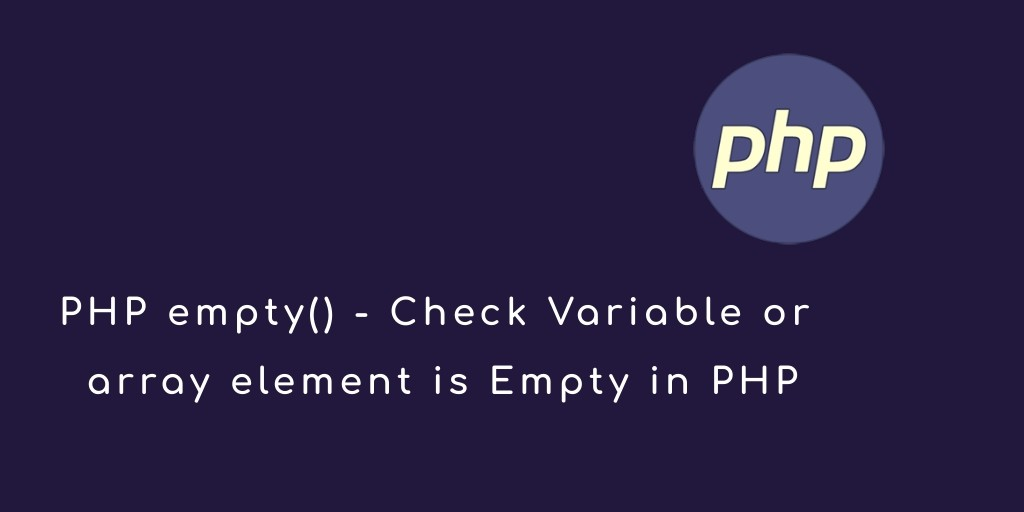Php 28-Jul-2021

### PHP empty() – Check Variable is Empty in PHP

empty() function in PHP. Here we will learn what is an empty function and how to use this function. Basically, empty() function can be used to check a variable is empty or not in PHP.

## empty() function in PHP

PHP empty() function is used to check whether if a variable is empty or not. It will return True if the given variable is empty and false. If it exists or a non-zero value or any value in it, it will return false.

### Syntax – empty() function

`empty(\$variable);`

In PHP empty funciton the given variables can be of different types like an integer, string or even an array etc.

### Example – empty function

Let’s take an example of the PHP empty() function. In the following example, we will declare a variable and given a null value.

<?php

//The empty function example
\$str = "";

if (empty(\$str)) {
echo "The variable is empty";
} else {
echo "The variable is not empty";
}

?>

#### Source code – PHP empty() function

<!DOCTYPE html>
<html>
<title>PHP empty() function is used to check whether a variable is empty or not. | PHP Tutorial</title>
<body>

<h4>To check a whether a variable is empty or not</h4>

<?php

//The empty function example
\$str = "";

if (empty(\$str)) {
echo "The variable is empty";
} else {
echo "The variable is not empty";
}
?>

</body>
</html>

### Second Example – emtpy() function

<?php

//The empty function example
\$str = 0;

if (empty(\$str)) {
echo "The variable is empty";
} else {
echo "The variable is not empty";
}

?>

Second Example Source code – empty() function

<!DOCTYPE html>
<html>
<title>PHP empty() function is used to check whether a variable is empty or not. | PHP Tutorial</title>
<body>

<h4>To check a whether a variable is empty or not</h4>

<?php

//The empty function example
\$str = 0;

if (empty(\$str)) {
echo "The variable is empty";
} else {
echo "The variable is not empty";
}

?>

</body>
</html>

### Empty() example with array elements

Let’s take a new example of empty() function with array elements. Here we will declare an array and assigned a value of the array elements. And here we will assign a 0 value of the third element of the array, remaining array elements assign a non zero value.

//The empty function example
\$arr = array();
\$arr["john"] = 100;
\$arr["mack"] = 50;
\$arr["rose"] = 0;

foreach(\$arr as \$key => \$value){
if (empty(\$value)){
echo "(This is empty) " . \$key . " = " .\$value ."<br />";
}
else{
echo "(This is not empty) " . \$key . " = " .\$value ."<br />";
}
}

Source Code – Empty() example with array elements

<!DOCTYPE html>
<html>
<title>PHP Empty() example with array elements | PHP Tutorial</title>
<body>

<h4>PHP Empty() example with array elements</h4>

<?php

//The empty function example
\$arr = array();
\$arr["john"] = 100;
\$arr["mack"] = 50;
\$arr["rose"] = 0;

foreach(\$arr as \$key => \$value){
if (empty(\$value)){
echo "(This is empty) " . \$key . " = " .\$value ."<br />";
}
else{
echo "(This is not empty) " . \$key . " = " .\$value ."<br />";
}
}
?>

</body>
</html>

### Why we use the empty function?

Sometimes, you need to check if a variable contains a value or not before proceeding to some action.

For example, you are taking the input values from the user in a web form. A few fields like Name, email, address are mandatory in the form. While you can check it at the client side by using the JavaScript, however, sometimes it may be disabled.

In that case, you may check the variable values by using the empty function to ensure that a user has entered some values or otherwise redirect the user back to the form page rather than proceeding to save the information in the database.

### How to use PHP empty function

Using the empty function is quite simple. You simply need to provide a variable name to check if it contains a value or is it is empty.

If your string contains no characters or it is like this:

`Str = “”;`

Then this will be considered as empty.

• Declared a variable is not defined or does not exist, it will be considered as empty.
• Sometimes, you have declared a variable and not given any value, this will still be considered as empty.
• If a Boolean variable is False, this is also considered as empty.
• The integer or float variable with 0 value are also considered as empty.
• A variable with NULL value is also taken as empty.
• An empty array is also taken as “empty”.